# Python | Pandas MultiIndex.names

Python is a great language for doing data analysis, primarily because of the fantastic ecosystem of data-centric python packages. Pandas is one of those packages and makes importing and analyzing data much easier.

Pandas` MultiIndex.names` attribute returns the names of levels in the MultiIndex.

Syntax: MultiIndex.names

Example #1: Use `MultiIndex.names` attribute to find the names of the levels in the MultiIndex.

 `# importing pandas as pd ` `import` `pandas as pd ` ` `  `# Creating the array ` `array ``=` `[[``1``, ``2``, ``3``], [``'Sharon'``, ``'Nick'``, ``'Bailey'``]] ` ` `  `# Print the array ` `print``(array) `

Output :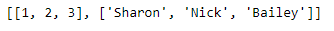Now let’s create the MultiIndex using this array

 `# Creating the MultiIndex ` `midx ``=` `pd.MultiIndex.from_arrays(array, names ``=``(``'Number'``, ``'Names'``)) ` ` `  `# Print the MultiIndex ` `print``(midx) `

Output :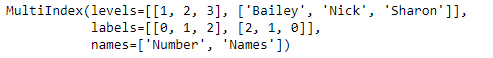Now we will find the names of all the levels in the MultiIndex.

 `# Print the names of the level in MultiIndex ` `midx.names `

Output :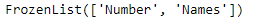As we can see in the output, midx has two levels and the name of the levels are ‘Number’ and ‘Names’.

Example #2: Use `MultiIndex.names` attribute to find the names of the levels in the given MultiIndex.

 `# importing pandas as pd ` `import` `pandas as pd ` ` `  `# Creating the array ` `array ``=``[[``1``, ``2``, ``3``], [``'Sharon'``, ``'Nick'``, ``'Bailey'``],  ` `           ``[``'Doctor'``, ``'Scientist'``, ``'Physicist'``]] ` ` `  `# Print the array ` `print``(array) `

Output :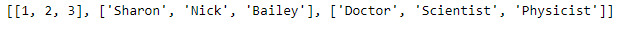Now let’s create the MultiIndex using this array

 `# Creating the MultiIndex ` `midx ``=` `pd.MultiIndex.from_arrays(array,  ` `   ``names ``=``(``'Ranking'``, ``'Names'``, ``'Profession'``)) ` ` `  `# Print the MultiIndex ` `print``(midx) `

Output :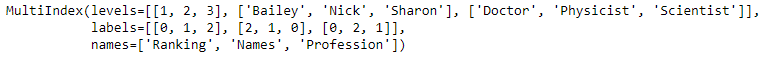Now we will find the names of all the levels in the MultiIndex.

 `# Print the names of the level in MultiIndex ` `midx.names `

Output :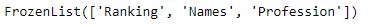As we can see in the output, midx has three levels and the name of the levels are ‘Number’, ‘Names’ and ‘Profession’.

My Personal Notes arrow_drop_upCheck out this Author's contributed articles.

If you like GeeksforGeeks and would like to contribute, you can also write an article using contribute.geeksforgeeks.org or mail your article to contribute@geeksforgeeks.org. See your article appearing on the GeeksforGeeks main page and help other Geeks.

Please Improve this article if you find anything incorrect by clicking on the "Improve Article" button below.

Article Tags :

Be the First to upvote.

Please write to us at contribute@geeksforgeeks.org to report any issue with the above content.